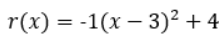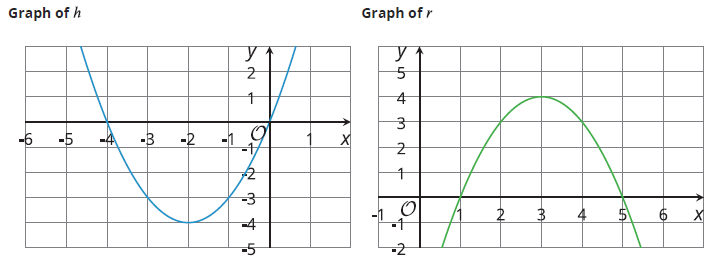# A.6.15.2 A Whole New Form

Here are two sets of equations for quadratic functions. In each column, the expressions that define the output are equivalent. f(x) = g(x) = h(x) and p(x) = q(x) = r(x) You may recognize 2 of the forms; the first row is standard form and the second row is factored form. The third row is a new form for today!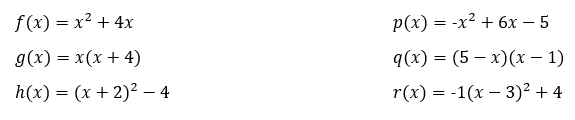The expression that defines h is written in vertex form. We can show that it is equivalent to the expression defining by expanding the expression: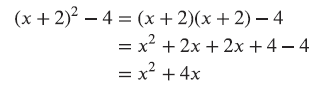Show that the expressions defining p(x), q(x), and r(x) are equivalent by multiplying out r(x).

Here are graphs representing the quadratic functions, they are written in vertex form. Vertex form contains the vertex (the point on the parabola that lies on the line of symmetry of the parabola - or what you can think of as the "middle" point). Notice the x-value is subtracted from x in the equation, so it has the opposite sign of the vertex. The y-value of the vertex has the same sign in the vertex point as in the equation. Vertex is the point (-2, -4) ﻿ ﻿ ﻿ ﻿ ﻿ ﻿ Vertex is the point (3, 4)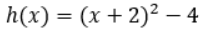﻿ ﻿ ﻿ ﻿ ﻿